# Python Program to Swap Two Variables

In this tutorial you will learn about the Python Program to Swap Two Variables and its application with practical example.

In this tutorial, we will learn to create a Python Program to Swap Two Variables using python Programming.

## Prerequisites

Before starting with this tutorial we assume that you are best aware of the following Python programming topics:

• Python Operator.
• Basic Input and Output
• Basic Python  programming.
• if-else statements in Python.
• Loops in Python.

## Swapping two variable

This is one the basic Program in any language, where  we  display the how operator work and also how the assigned values in variables and use or operators in it.

## Python Program to Swap Two Variables

In this program we will swap two variables value using third variable .First we would declared and initialized the required variables. Next, we would prompt user to input two values. Later we swap their values.

## Output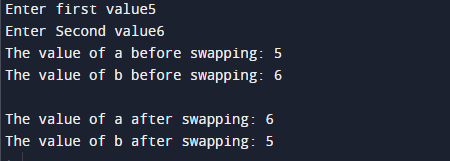In our program, we have first declared and initialized a set variables required in the program.

• a= it will hold first value from user.
• b= it will hold second value from user.
• c= it will hols temporary value of variables.

First of all  we would declare variable and take a value from user and assign in variable ‘a‘ and ‘b‘  respectively.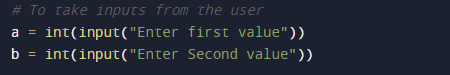In our program, we use the temporary variable ‘c‘ which will hold the value of ‘a‘ temporarily. then we put the value of ‘b‘ in ‘a‘ and after that ‘c’in ‘b’ .Which s shown in image below.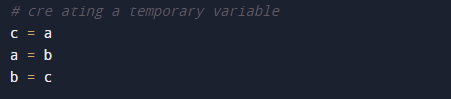we can also swap values of variables using arithmetic operations to do the same without using third variable.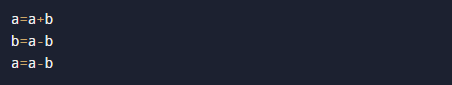and also there is a simple method to swap variables. The code below does the same as above but without the use of any temporary  variable or arithmetic operator.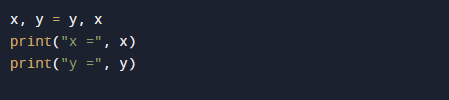Finally we will print the swapped value from user.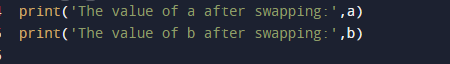areIn this tutorial we have learn about the Python Program to Swap Two Variables and its application with practical example. I hope you will like this tutorial.